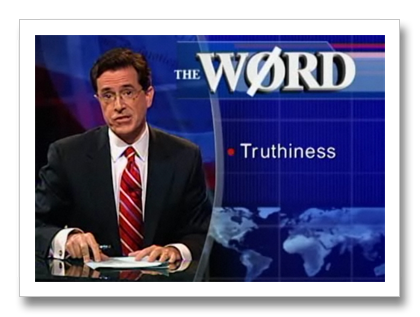## Conditionals

Ref. WGR Chapter 6, Control-flow techniques

In this section we cover truth, falsiness, and conditions.

## Truthiness and Falsiness(*not* in the Steven Colbert sense)

• Truthy things make "if" succeed
• Falsey things make "if" fail

## Falsey Things

• `nil`
• `false`
• that's it!

## Truthy Things

• `true`
• `0`
• `""`
• `"false"`
• pretty much anything else

## `if`

``````if condition then
statement
end
``````
• if `condition` is truthy then execute `statement`

• `then` is optional, so you normally use

``````if condition
statement
end
``````

## one-line `if`

``````if condition then statement end

if condition; statement; end

statement if condition
``````

## `else`

``````if condition
statement1
else
statement2
end
``````
• if `condition` is truthy then execute `statement1`
• if `condition` is falsy then execute `statement2`

## `elsif`

``````if condition1
statement1
elsif condition2
statement2
else
statement3
end
``````

it's spelled `elsif` not `elseif` or `else if`

## `not`

``````if x == 2
puts "two"
end

if not x == 2
puts "not two"
end
``````

## `not` vs. `!`

``````if x == 2
puts "two"
end

if not x == 2
puts "not two"
end

if !x == 2
puts "not what you think!"
end
``````

## `!` is clingy

The bang operator binds very tightly

``````not x == 2  #=> not (x == 2)
!x == 2     #=> (!x) == 2
``````

so that actually means ```ruby if (!x) == 2 ```

and assuming `x` is a number, `!x` will always be `false`

## `!` gotcha solved

``````    if !(x == 2)
puts "not two"
end
``````
• Moral: use `not` in conditions
• or use `unless`
• or use `!=`
• or use a lot of parentheses
• they're free!

## `!=`

• "bang equal" means "not equal"
• `x != 2` is equivalent to `!(x == 2)`

## unless

• `unless` means "if not"

``````puts "night" unless day
``````
• it can make your code read better

``````wear_sweater unless summer? or wearing_sweater?
``````
• it can also make your code read worse

``````button.hide unless not button.disabled?
``````
• never use `unless` with `not` or `else`

• unless you really know what you're doing `:-)`
• double negatives are not unconfusing

## Logic is hard

Statement Value
not nil true
not 0 false
!0 false
0 == false false
nil == false false
y = false false
y == false true
x = 0 0
"foo" if (x = 0) "foo"
x == y false
x != y true
!x==y true
not x==y true

## Comparison or Assignment?

Language Statement Purpose
mathematics X = 2 comparison
Basic LET X = 2 assignment
Fortran X = 2 assignment :-(
X == 2 comparison
Algol, Pascal X := 2 assignment :-)
X = 2 comparison :-)

"A notorious example for a bad idea was the choice of the equal sign to denote assignment. It goes back to Fortran in 1957 and has blindly been copied by armies of language designers. Why is it a bad idea? Because it overthrows a century old tradition to let "=" denote a comparison for equality, a predicate which is either true or false. But Fortran made it to mean assignment, the enforcing of equality. In this case, the operands are on unequal footing: The left operand (a variable) is to be made equal to the right operand (an expression). x = y does not mean the same thing as y = x."

Niklaus Wirth, Good Ideas, Through the Looking Glass (2005)

## assignment in conditionals

• `if x = 1` gives a warning, since it will always be true
• `if x = y` gives no warning, since you might mean it

• it still looks funny
• it can be useful, e.g.

``````if (last_name = person.family_name)
# person.family_name is not nil, so...
puts last_name
end
``````

## `case`

``````case var
when value1
puts "var is sorta value1"
when value2, value3
puts "var is sorta value2 or maybe value3"
else
puts "var is weird"
end
``````

## threequal

• case comparison uses the `===` operator
• aka "threequal"
• it's normally the same as `==` but can be overridden
• e.g. for Class, `===` also means `is_a?`, so you can do this:
``````case input
when Fixnum
input
when String
input.to_i
when Array
input.first.to_i
end
``````

## threequal transitivity gotcha

``````>> Fixnum === 1
=> true
>> 1 === Fixnum
=> false
``````

### Solution: use `is_a?` instead:

``````>> 1.is_a? Fixnum
=> true
``````

## a note on Boolean

There is no Boolean class in Ruby

Only `TrueClass` and `FalseClass`

## `!!`

• `!!` converts anything into either true (if it's truthy) or false (if it's falsey)
• but cool Rubyists never use `!!`
• they're down with truthiness

## or-equals

``````def name
@name ||= "Anonymous"
end
``````
• Means "if `@name` has a value, cool, but otherwise make it `'Anonymous'`"
• Relies on "logical or" and "nil is falsey" semantics
• There's also "plus-equals" (`+=`) and so forth

## or-equals expanded

These are equivalent:

@name ||= "Alex"

## as a boolean expression

@name || (@name = "Alex")

## with an if statement

if (@name != nil) @name else @name = "Alex" end